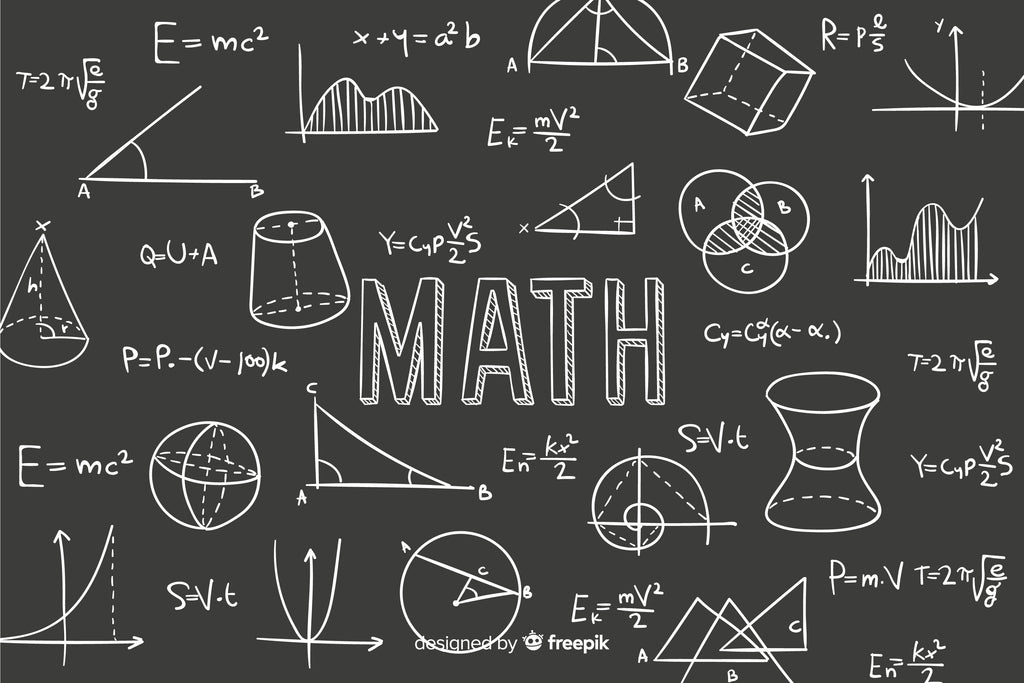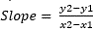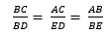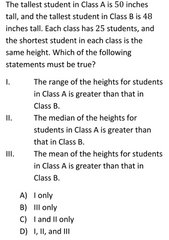# Common SAT Math problems and how to solve them: Part (II)Thinking of taking SATs but not sure what to expect in the SAT Math

Currently preparing for SAT Math and want to know which skills are a must to ace the test?

Finished preparing for SATs and want to quickly glance through some common Math problems?

If you don’t know it already, SAT Math tests students in different areas of mathematics, ranging from algebra and arithmetic to statistics, geometry and trigonometry.

This article takes a closer look at such questions and discusses the best approach to solve them following Part (I).

### 1. Functions and Graphs – Formulating and Solving EquationsThe question asks you to find the value of v, which as we can see is the x-coordinate of the point of intersection of the line and the parabola.

To find the point of intersection, we need to solve the two equations representing the line and the parabola.

But then, the equations of both the line and the parabola are not given. So, the first step is to formulate their respective equations.

The parabola has the vertex (4, 19). We know that the equation of a parabola in the vertex form is y = A(x – h)2 + k, where (h, k) represents the vertex.

Substituting h = 4 and k = 19, the equation becomes y = A(x – 4)2 + 19. Now, the only information required to have a full equation of the parabola is A.

Since (0, 3) passes through the parabola, substituting x = 0 and y = 3 should satisfy the equation, which eventually yields A = -1.

Thus, the equation of the parabola is y = -(x – 4)2 + 19. (Sanity check: since the vertex is the maximum point of the parabola, A being negative makes sense.)

To find the equation of the line, we need two information: y-intercept and slope. Since the line intersects Y-axis at (0, -9), we know that the y-intercept is -9. To calculate the slope, we can make use of the two coordinates: (0, -9) and (2, -1).

Using, we find that slope is 4. The equation of the line is y = 4x – 9. (Sanity check: since the line is ascending, the positive slope makes sense)

#### Now to find the point of intersection, we have to solve the system of the following two equations:

y = -(x – 4)2 + 19

y = 4x – 9

Substituting y from the second equation to the first equation, we get

4x – 9 = -(x – 4)2 + 19

Moving every term in the right hand side to the left hand side, we get

(x – 4)2 + 4x – 9 – 19 = 0

• x2 – 8x + 16 + 4x – 28 = 0
• x2 – 4x – 12 = 0
• x2 – 6x + 2x – 12 = 0
• x (x – 6) + 2(x – 6) = 0
• (x + 2) (x – 6) = 0

This means x equals to either -2 or 6. Since the x we are looking for lies in the first quadrant, it must be positive. Hence, the value of v (x-coordinate of the point of intersection) is 6.

### 2. Trigonometry – Using SOH CAH TOA in Right TriangleThese kinds of questions test your ability to use the definition of trigonometric functions to connect angle with the sides of a right triangle. If you aren’t already aware, remind yourself of the following definitions:Image Courtesy: hpmusuem.org

The mnemonic “SOH CAH TOH” is often a good way to memorize these definitions. SOH, for example, stands for Sin, Opposite, Hypotenuse, meaning Sin θ =.

In this question, we are given that tan C = 3/4. This means the ratio of Opposite to Adjacent is 3 to 4. The side opposite to angle C of the triangle ABC is AB, and the side adjacent to angle C is AC. Thus,This implies AC = 4/3 AB.

Now, in triangle ABC, using Pythagoras theorem, we know that:

AB2 + AC2 = BC2

> AB2 + (4/3 AB)2 = 152

> AB = 9

Thus, the answer is 9, option A.

### 3. Geometry – Calculating Side Lengths using Similar TrianglesThese kinds of questions test your ability to apply the concepts of similar triangles to determine the length or ratio of lengths of a triangle.

The zeroth step to approaching these kind of problems-- with diagrams containing multiple triangles in such configurations-- is suspecting that the question involves identifying similar triangles.

In this figure, if we analyse triangle BDE and triangle ABC, the following are true:

∠ BED = ∠ BAC = 900

∠ EBD = ∠ ABC [Both are the same angles, hence equal in measurement]

> ∠ EDB = ∠ ACB [If two angles of triangles are equal, the third angle should also be equal as all angles of a triangle add up to 1800.]

Thus, by AAA axiom, we know that triangle BDE and triangle ABC are similar. This means their corresponding sides are proportional, i.e.Since we know AB = 12, BE = 5, and that AC = 2 DE + 1, we can use the equality of the second and third ratio as:Thus, the length of DE is 5/2, which is option B.

4. Statistics – Central Tendency and Data DispersionCollegeboard often tests in-depth understanding of measures of central tendency and data dispersion using these kinds of questions.

Here,

 Class A Class B Shortest student = same height as that of class B Shortest student = same height as that of class A Tallest student = 50 inches Tallest student = 48 inches

Since Range = Largest value – Smallest value, we can deduce that the range of heights of Class A must be greater than that of Class B. Hence statement I is correct.

The median is the middle value of the data distribution. In this case, since the number of students (n) = 25. The position of median is= 13. Hence, if the heights of 25 students are arranged in an ascending or descending order, the median height will be the 13th value.

The tallest student being of different heights in Class A and B does not affect the median as the tallest value is the extreme value, not the middle (‘median’) value.

This means, whatever the values of the extreme heights (tallest and shortest), there can be a scenario where the median (middle value) of class A can be either greater than, or equal to, or less than that of class B.

Hence, statement II could be true in some circumstances, but it is not necessary that it “must be true” as the question asks. So statement II is wrong.

The mean is the arithmetic average of all the values in the data. Every data value contributes to the overall average of the data.

Depending on how high or low all the data values are, the mean height of Class A students can be either greater than, or equal to, or less than that of Class B.

This means knowing that shortest values are same and the largest values are different, is insufficient to conclude which mean value is greater. Hence, statement III could be true in some circumstances, but it is not necessary that it “must be true” as the question asks. So, statement III is also wrong.

Thus, the answer is option A.

Recent posts